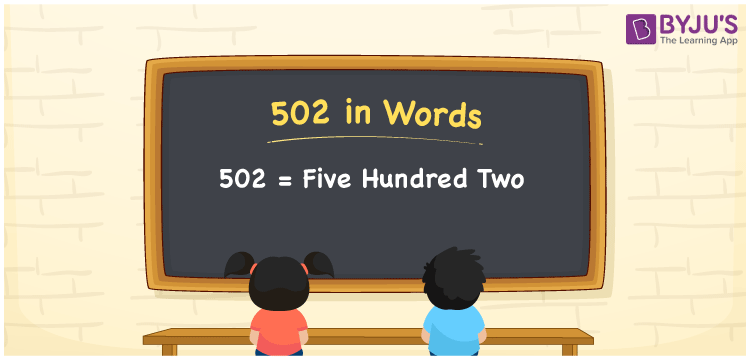# 502 in Words

502 in words is written as Five hundred two. In both the International System of Numerals and the Indian System of Numerals, 502 is written as Five hundred two. The number 502 is a Cardinal Number as it describes some quantity. For example, “There are 502 pages in that book”.

 502 in Words Five hundred two Five hundred two in Number 502

## 502 in English Words

We write 502 in Words using the letters of the English alphabet. Therefore, we read 502 in English as “Five hundred two.”## How to Write 502 in Words?

To write 502 in words, we shall use the place value chart. In the place value chart, write 2 in the ones, 0 in the tens, and 5 in the hundreds. Now let us make a place value chart to write the number 502 in words.

 Hundreds Tens Ones 5 0 2

Thus, we can write the expanded form as

5 × Hundred + 0 × Ten + 2 × One

= 5 × 100 + 0 × 10 + 2 × 1

= 500 + 0 + 2

= 502

= Five hundred two.

502 is a natural number which is the successor of 501 and the predecessor of 503.

502 in words – Five hundred two

• Is 502 an odd number? – No
• Is 502 an even number? – Yes
• Is 502 a perfect square number? – No
• Is 502 a perfect cube number? – No
• Is 502 a prime number? – No
• Is 502 a composite number? – Yes

## Frequently Asked Questions on 502 in Words

Q1

### How to write 502 in words?

502 in words is written as Five hundred two.
Q2

### How to write 502 in words in the International and Indian System of Numerals?

In both the systems of numerals, 502 is written as Five hundred two.
Q3

### How to write 502 in a place value chart?

In the place value chart, write 2 in ones, 0 in tens, and then 5 in the hundreds place.